Algebra 2 3-2 Complete Lesson: Solving Systems Algebraically
starstarstarstarstarstarstarstarstarstar
4.5 (1 rating)
by Matthew Richardson
| 25 Questions
Note from the author:
A complete formative lesson with embedded slideshow, mini lecture screencasts, checks for understanding, practice items, mixed review, and reflection. I create these assignments to supplement each lesson of Pearson's Common Core Edition Algebra 1, Algebra 2, and Geometry courses. See also mathquest.net and twitter.com/mathquestEDU.The outlined content above was added from outside of Formative.1
1
10 pts
Solve It! What whole dollar amount of one-day sales would make it more worthwhile to work at Store B?
Any amount over \$511
Any amount over \$215
Any amount over \$171
Any amount over \$313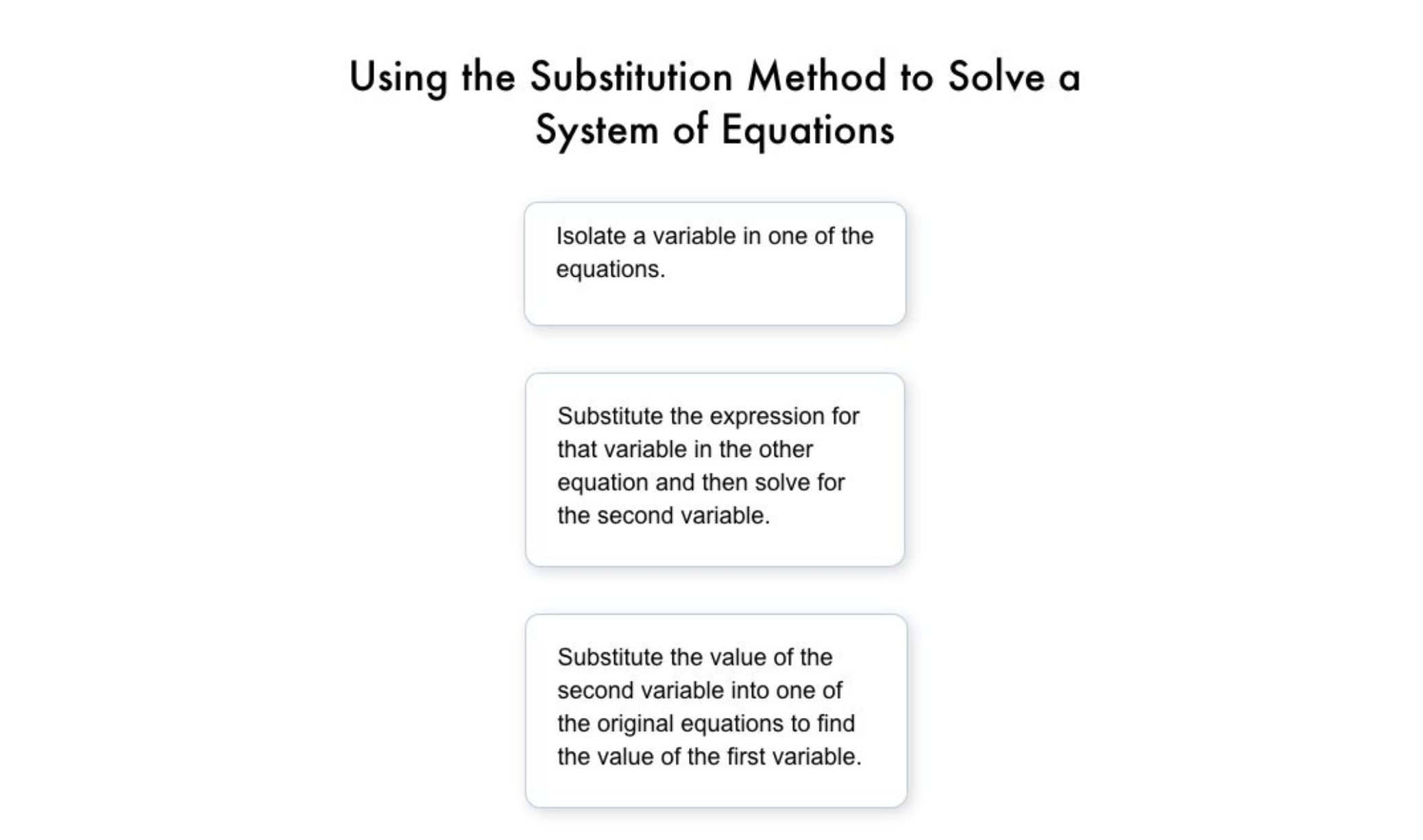2
2
10 pts
Problem 1 Got It?
A
B
C
D3
3
10 pts
Problem 2 Got It?
A
B
C
D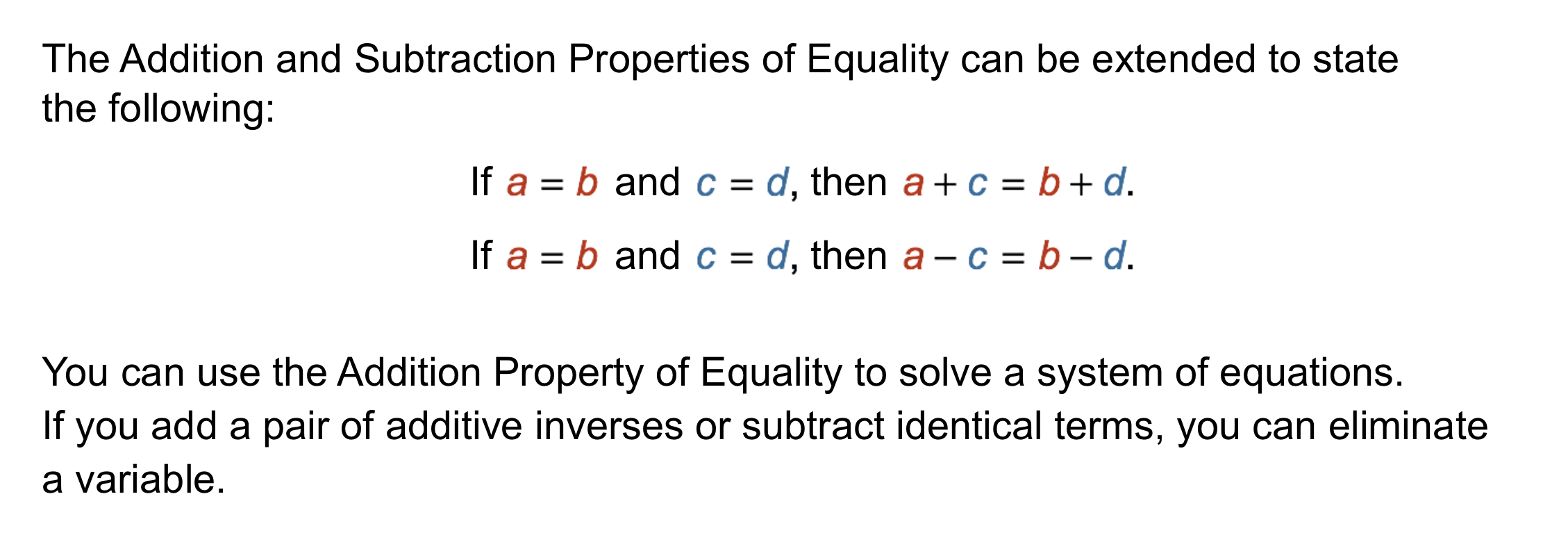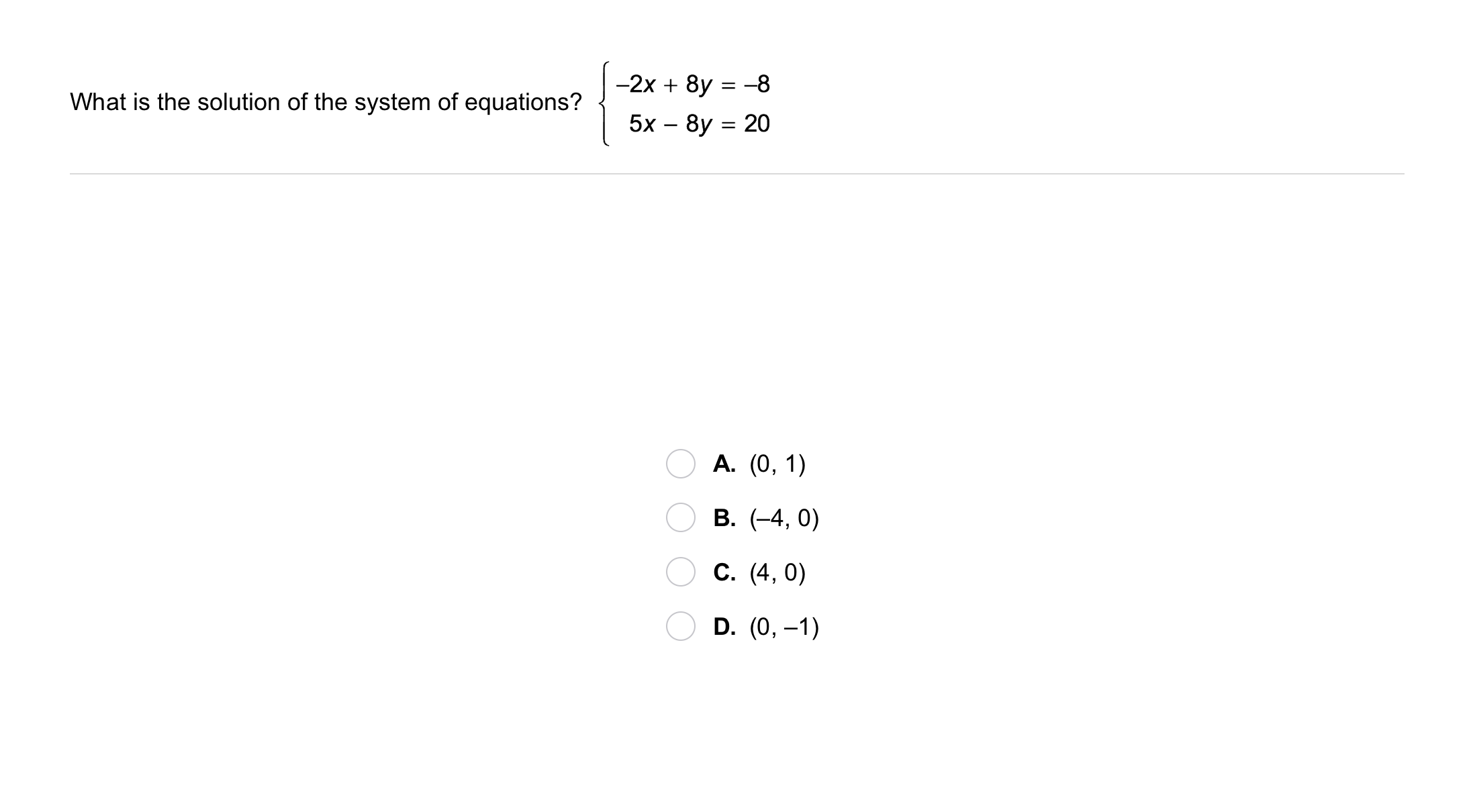4
4
10 pts
Problem 3 Got It?
A
B
C
D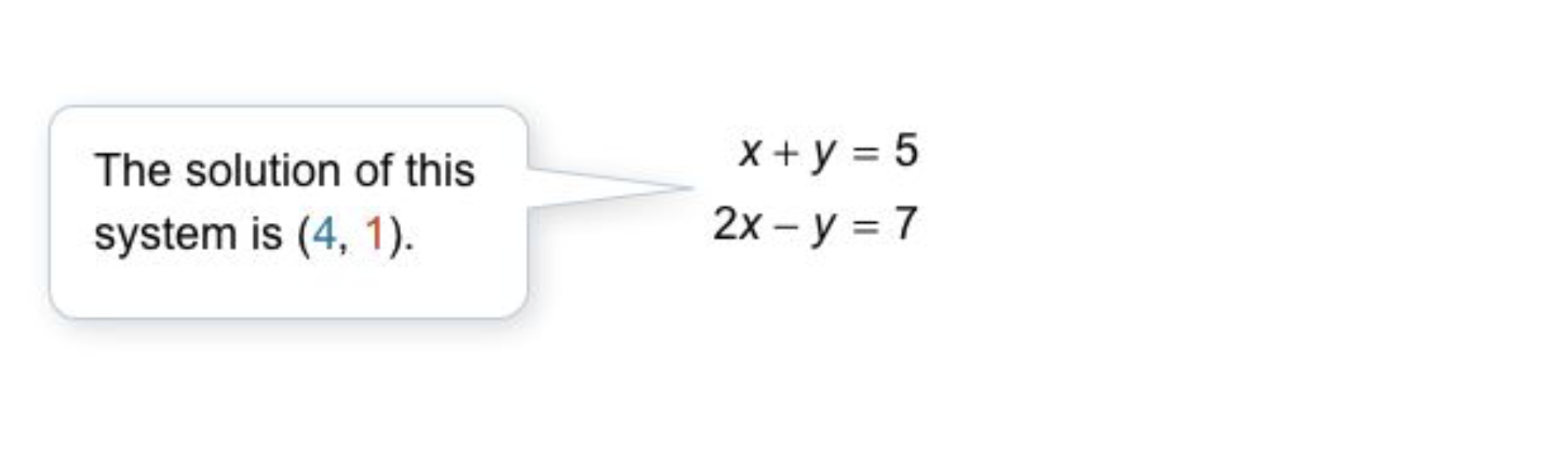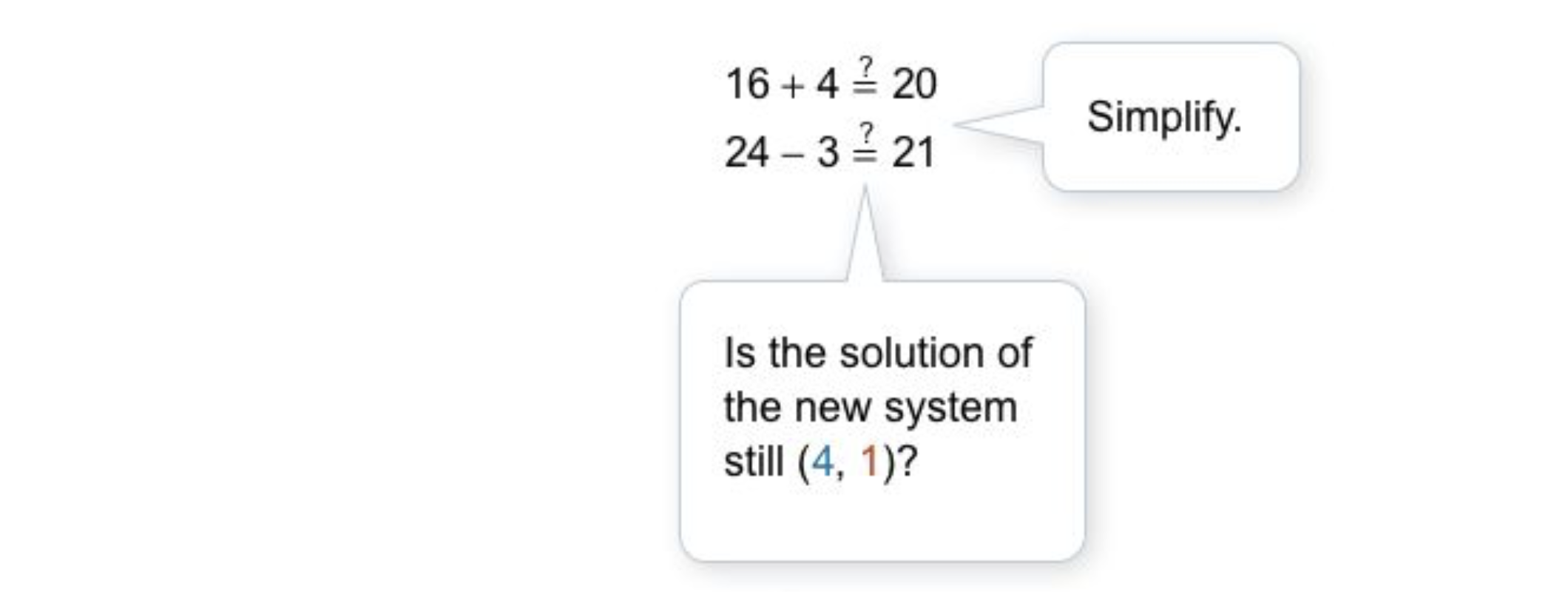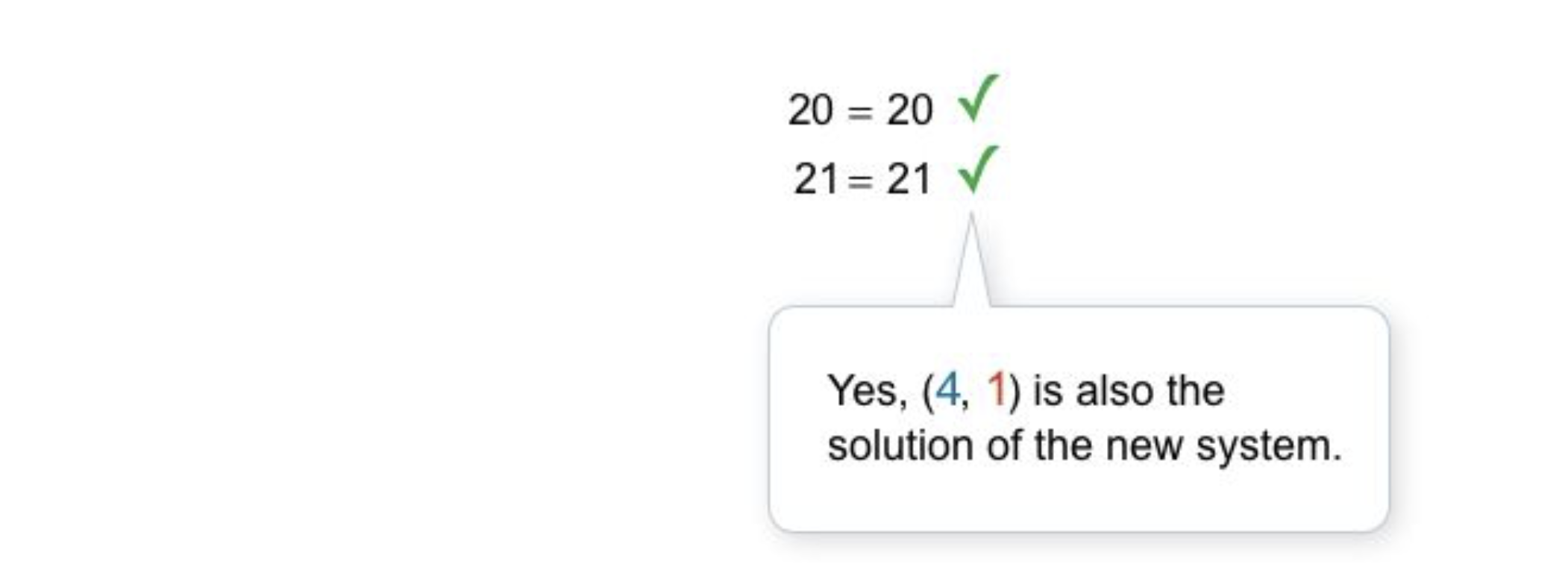5
10 pts
Problem 4 Got It? What is the solution of this system of equations?

(5, 2)
(-2, 3)
(-3, 2)
(3, -5)6
6
10 pts
Problem 5 Got It?
A
B
C
D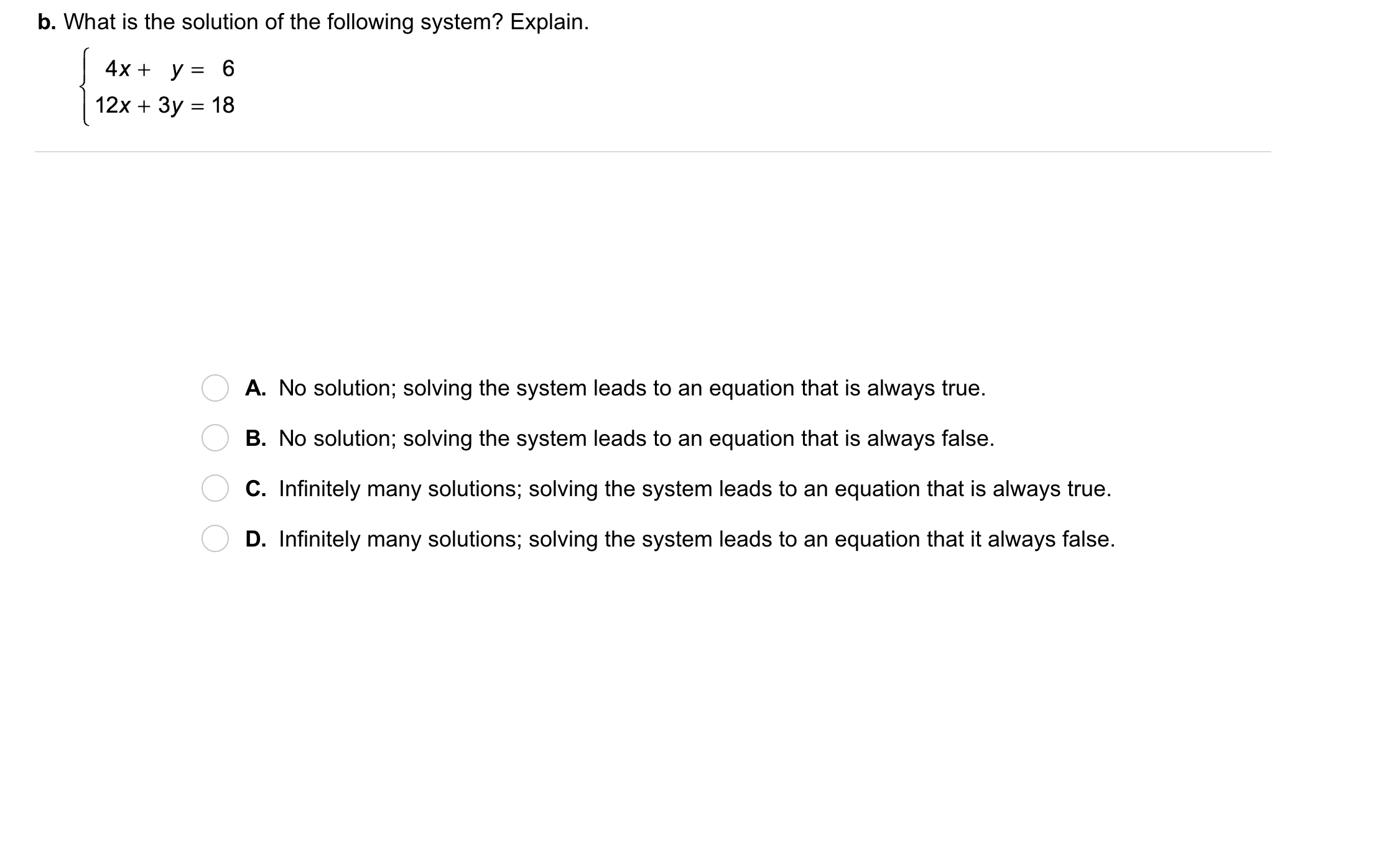7
7
10 pts
Problem 5 Got It?
A
B
C
D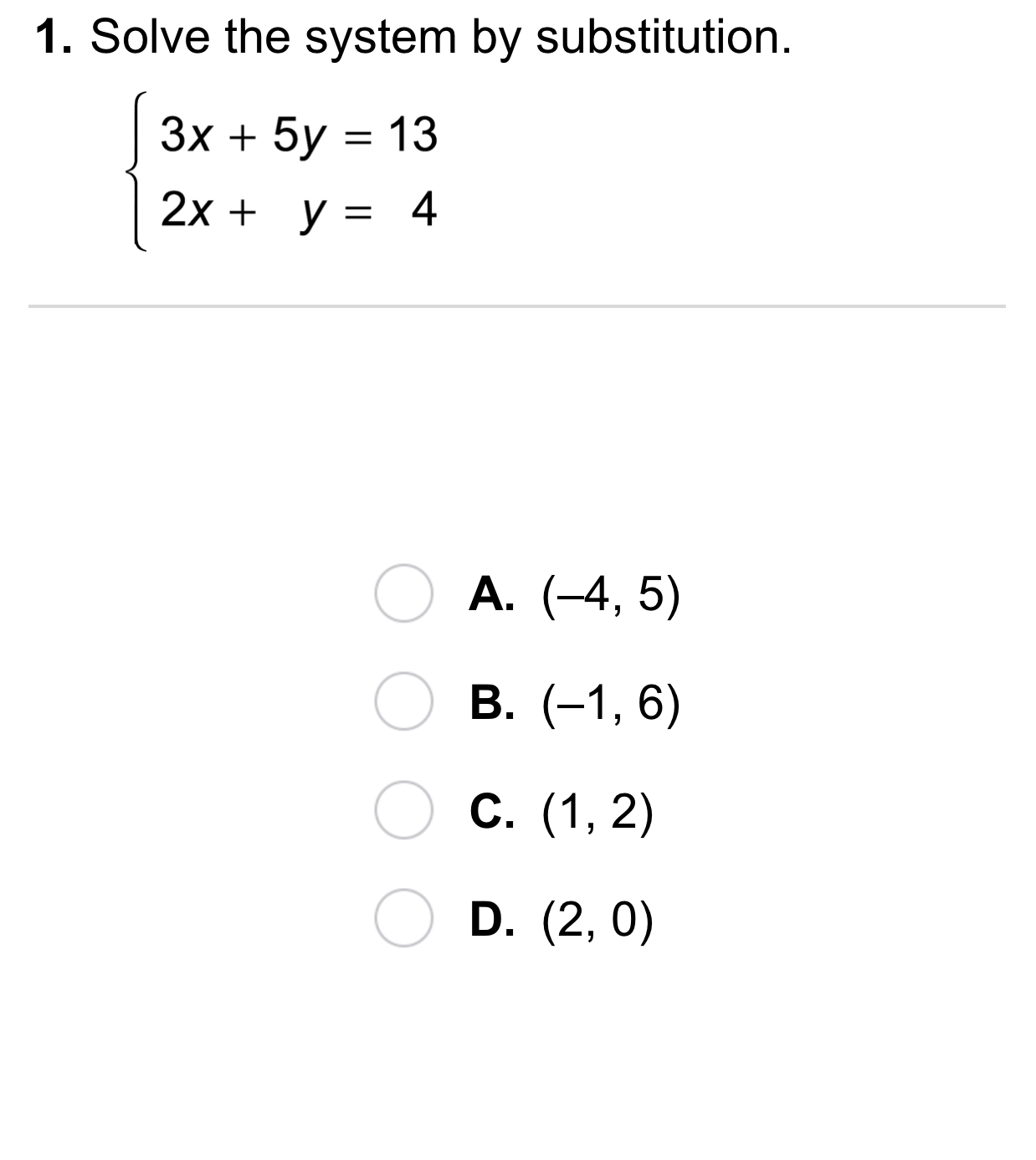8
8
10 pts
A
B
C
D9
9
10 pts
A
B
C
D10
10
10 pts
A
B
C
D11
11
10 pts
A
B
C
D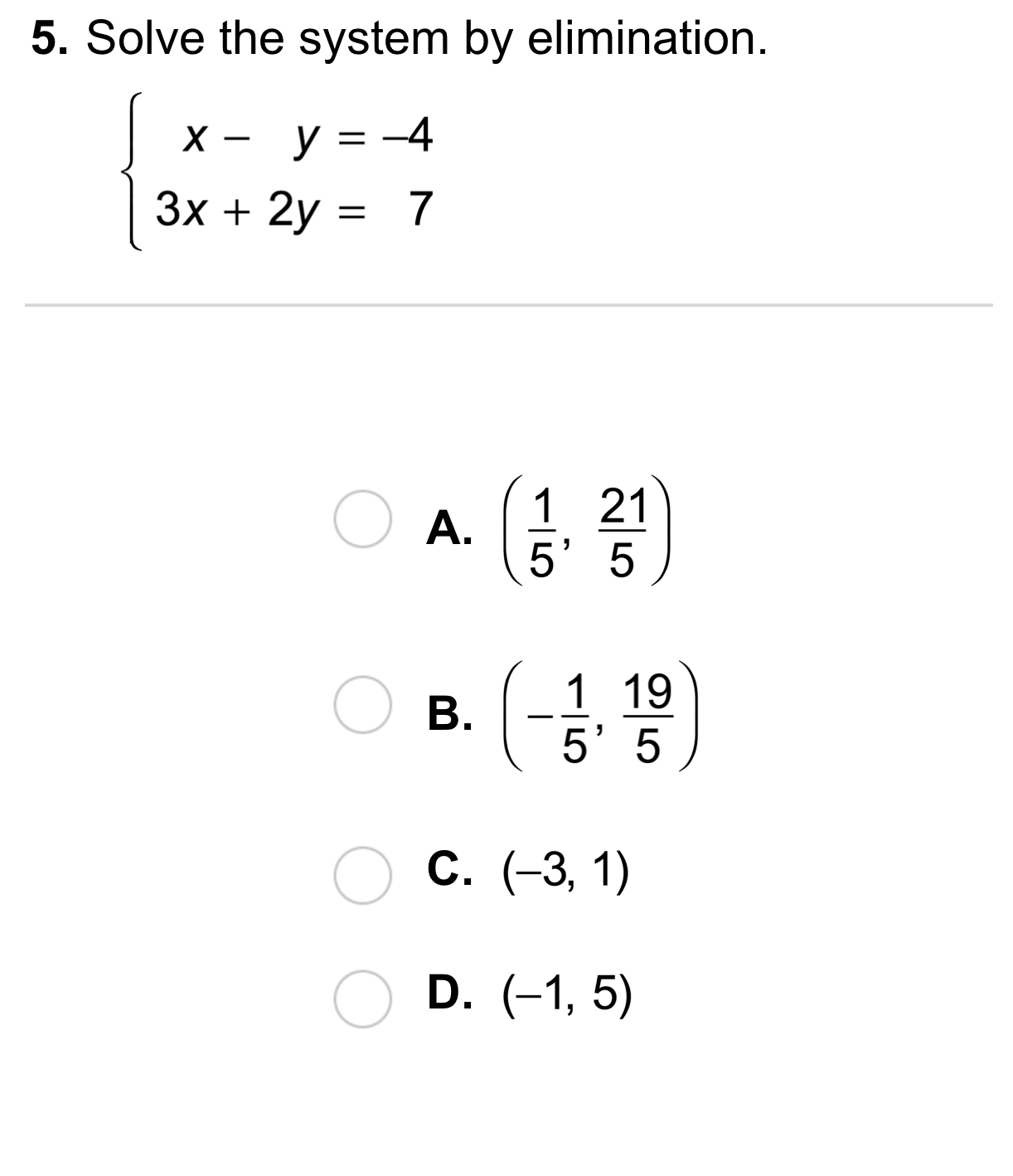12
12
10 pts
A
B
C
D13
13
10 pts
A
B
C
D14
10 pts
Vocabulary: Give an example of two equivalent systems.
16
20 pts
Writing: A cafe sells a regular cup of coffee for \$1 and a large cup for \$1.50. Melissa and her friend buy 5 cups of coffee and spend a total of \$6. Explain how to write and solve a system of equations to find the number of large cups of coffee they bought. Use the canvas to explain. You are not required to solve the system of questions, but your response should include the system and a narrative explaining how you set it up.17
10 pts
Review Lesson 3-1: Solve the system by graphing on the canvas.
18
10 pts
Review Lesson 3-1: Solve the same system using the Desmos graphing utility. Zoom and pan your graph to establish an appropraite viewing window. You may edit your response to the previous item, if needed.
19
10 pts
Review Lesson 2-1: Use the vertical line test to determine whether each graph represents a function. Categorize each graph appropriately.

• Is a function (passes the vertical line test)
• Is not a function (fails the vertical line test)20
10 pts
Vocabulary Review: Group the equations that are in standard form.

• Standard Form
21
10 pts
Vocabulary Review: Write the equation in standard form.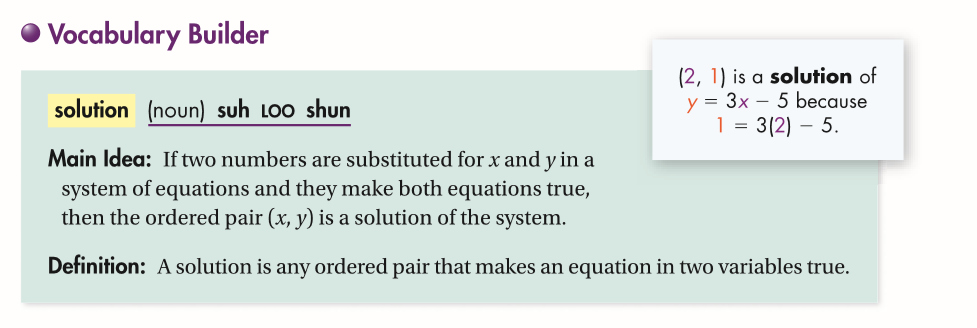22
10 pts
Use Your Vocabulary: Pair up x- and y-values to form two different solutions of the equation.

• x = 0
• x = 1
• y = -2
• y = -7
• y = 3
23
10 pts
Use Your Vocabulary: Consider the system below. Categorize each statement regarding the system as true or false.
• The system has a unique solution.
• The system has infinitely many solutions.
• The system has no solution.
• The solution is (0, 0).
• The solution of a system can be found by graphing the equations of the system.
• True
• False
24
100 pts
Notes: Take a clear picture or screenshot of your Cornell notes for this lesson. Upload it to the canvas. Zoom and pan as needed.

For a refresher on the Cornell note-taking system, click here.
25
10 pts
Reflection: Math Success
Add to my formatives list

Formative uses cookies to allow us to better understand how the site is used. By continuing to use this site, you consent to the Terms of Service and Privacy Policy.